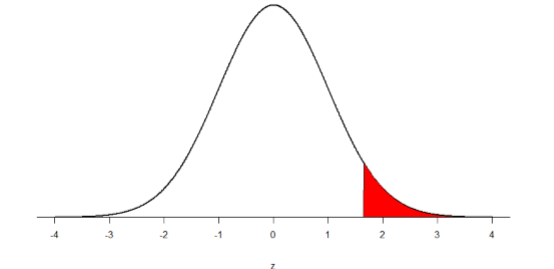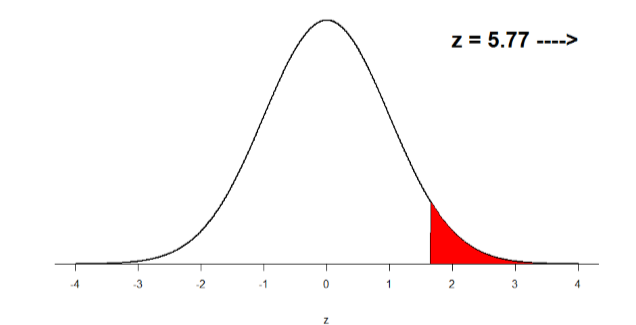# 8.9: Office Temperature

$$\newcommand{\vecs}{\overset { \rightharpoonup} {\mathbf{#1}} }$$ $$\newcommand{\vecd}{\overset{-\!-\!\rightharpoonup}{\vphantom{a}\smash {#1}}}$$$$\newcommand{\id}{\mathrm{id}}$$ $$\newcommand{\Span}{\mathrm{span}}$$ $$\newcommand{\kernel}{\mathrm{null}\,}$$ $$\newcommand{\range}{\mathrm{range}\,}$$ $$\newcommand{\RealPart}{\mathrm{Re}}$$ $$\newcommand{\ImaginaryPart}{\mathrm{Im}}$$ $$\newcommand{\Argument}{\mathrm{Arg}}$$ $$\newcommand{\norm}{\| #1 \|}$$ $$\newcommand{\inner}{\langle #1, #2 \rangle}$$ $$\newcommand{\Span}{\mathrm{span}}$$ $$\newcommand{\id}{\mathrm{id}}$$ $$\newcommand{\Span}{\mathrm{span}}$$ $$\newcommand{\kernel}{\mathrm{null}\,}$$ $$\newcommand{\range}{\mathrm{range}\,}$$ $$\newcommand{\RealPart}{\mathrm{Re}}$$ $$\newcommand{\ImaginaryPart}{\mathrm{Im}}$$ $$\newcommand{\Argument}{\mathrm{Arg}}$$ $$\newcommand{\norm}{\| #1 \|}$$ $$\newcommand{\inner}{\langle #1, #2 \rangle}$$ $$\newcommand{\Span}{\mathrm{span}}$$$$\newcommand{\AA}{\unicode[.8,0]{x212B}}$$

Let’s do another example to solidify our understanding. Let’s say that the office building you work in is supposed to be kept at 74 degree Fahrenheit but is allowed to vary by 1 degree in either direction. You suspect that, as a cost saving measure, the temperature was secretly set higher. You set up a formal way to test your hypothesis.

Step 1: State the Hypotheses

You start by laying out the null hypothesis:

$$H_0$$: The average building temperature is not higher than claimed

$$H_0: \mu \leq 74$$

Next you state the alternative hypothesis. You have reason to suspect a specific direction of change, so you make a one-tailed test:

$$H_A$$: The average building temperature is higher than claimed

$$\mathrm{H}_{\mathrm{A}}: \mu>74$$

Step 2: Find the Critical Values

You know that the most common level of significance is $$α$$ = 0.05, so you keep that the same and know that the critical value for a one-tailed $$z$$-test is $$z*$$ = 1.645. To keep track of the directionality of the test and rejection region, you draw out your distribution:Figure $$\PageIndex{1}$$: Rejection region

Step 3: Calculate the Test Statistic

Now that you have everything set up, you spend one week collecting temperature data:

Table $$\PageIndex{1}$$: Temperature data for a week
Day Temperature
Monday 77
Tuesday 76
Wednesday 74
Thursday 78
Friday 78

You calculate the average of these scores to be $$M$$= 76.6 degrees. You use this to calculate the test statistic, using $$μ$$ = 74 (the supposed average temperature), $$σ$$ = 1.00 (how much the temperature should vary), and $$n$$ = 5 (how many data points you collected):

$z=\dfrac{76.60-74.00}{1.00 / \sqrt{5}}=\dfrac{2.60}{0.45}=5.78 \nonumber$

This value falls so far into the tail that it cannot even be plotted on the distribution!Figure $$\PageIndex{2}$$: Obtained $$z$$-statistic

Step 4: Make the Decision

You compare your obtained $$z$$-statistic, $$z$$ = 5.77, to the critical value, $$z*$$ = 1.645, and find that $$z > z*$$. Therefore you reject the null hypothesis, concluding:

Based on 5 observations, the average temperature ($$M$$= 76.6 degrees) is statistically significantly higher than it is supposed to be, $$z$$ = 5.77, $$p$$ < .05.

Because the result is significant, you also calculate an effect size:

$d=\dfrac{76.60-74.00}{1.00}=\dfrac{2.60}{1.00}=2.60 \nonumber$

The effect size you calculate is definitely large, meaning someone has some explaining to do!